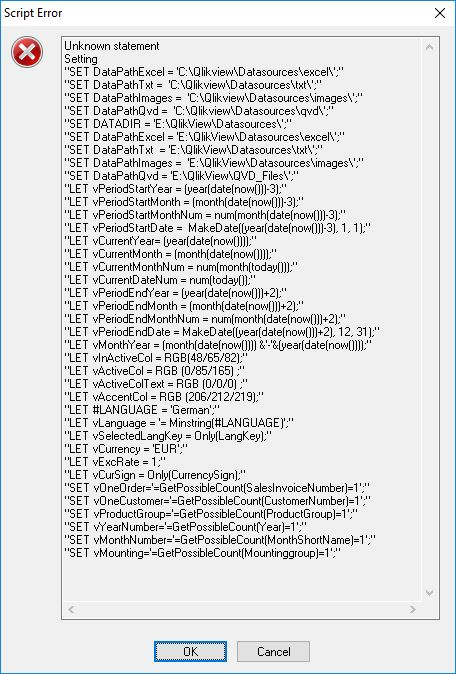# QlikView Scripting

Discussion Board for collaboration on QlikView Scripting.

Contributor

## Store global settings into a txt-file for include-statement

Hello,

We want to maintain the base of our Qlikviewdocument-settings on one central location, so in

our DWH we have created a file GlobalVariables with settings for those Qlikview Documents.

In a Qlikview Document (see attach document) I create a table GlobalVariables:

GlobalVariables:
VariableName,
VariableContent,
VariableDescription;
SQL SELECT *
FROM "QlikView_Repository".dbo.GlobalVariables;

ToTXTfile:
VariableContent   as Setting
Resident GlobalVariables;

This table ToTXTfile I store as a TXT-file:

Store ToTXTfile Into \$(DataPathTxt)GlobalVariables.txt (txt, delimiter is ';');

The txt-file I get, I cannot use in an included statement like:

\$(Include=c:\qlikview\datasources\txt\globalvariables.txt);

because I get load-failures and so the txt-file is not like expected:

Setting

"SET DataPathExcel = 'C:\Qlikview\Datasources\excel\';"

"SET DataPathTxt =  'C:\Qlikview\Datasources\txt\';"

"SET DataPathImages =  'C:\Qlikview\Datasources\images\';"

"SET DataPathQvd =  'C:\Qlikview\Datasources\qvd\';"

"SET DataPathExcel = 'E:\QlikView\Datasources\excel\';"

"SET DataPathTxt  = 'E:\QlikView\Datasources\txt\';"

"SET DataPathImages =  'E:\QlikView\Datasources\images\';"

"SET DataPathQvd = 'E:\QlikView\QVD_Files\';"

"LET vPeriodStartYear = (year(date(now()))-3);"

"LET vPeriodStartMonth = (month(date(now()))-3);"

"LET vPeriodStartMonthNum = num(month(date(now()))-3);"

"LET vPeriodStartDate =  MakeDate((year(date(now()))-3), 1, 1);"

"LET vCurrentYear= (year(date(now())));"

"LET vCurrentMonth = (month(date(now())));"

"LET vCurrentMonthNum = num(month(today()));"

"LET vCurrentDateNum = num(today());"

"LET vPeriodEndYear = (year(date(now()))+2);"

"LET vPeriodEndMonth = (month(date(now()))+2);"

"LET vPeriodEndMonthNum = num(month(date(now()))+2);"

"LET vPeriodEndDate = MakeDate((year(date(now()))+2), 12, 31);"

"LET vMonthYear = (month(date(now()))) &'-'&(year(date(now())));"

"LET vInActiveCol = RGB(48/65/82);"

"LET vActiveCol = RGB (0/85/165) ;"

"LET vActiveColText = RGB (0/0/0) ;"

"LET vAccentCol = RGB (206/212/219);"

"LET #LANGUAGE = 'German';"

"LET vLanguage = '= Minstring(#LANGUAGE)';"

"LET vSelectedLangKey = Only(LangKey);"

"LET vCurrency = 'EUR';"

"LET vExcRate = 1;"

"LET vCurSign = Only(CurrencySign);"

"SET vOneOrder='=GetPossibleCount(SalesInvoiceNumber)=1';"

"SET vOneCustomer='=GetPossibleCount(CustomerNumber)=1';"

"SET vProductGroup='=GetPossibleCount(ProductGroup)=1';"

"SET vYearNumber='=GetPossibleCount(Year)=1';"

"SET vMonthNumber='=GetPossibleCount(MonthShortName)=1';"

"SET vMounting='=GetPossibleCount(Mountinggroup)=1';"

When I use the txt-file in n included statement and give a reload, the reload has problems with the VariableContent and "

My questions:

1. Is it possible to create a txt-file which can be used in an included statement?
2. When Yes: what should be changed in the store statement to get the right txt-file for the include statement?
3. When NO: is there another possible solution to get the settings from the DWH into a Qlikview Document and use them as Settings not as a Table?
4 Replies
Honored Contributor II

## Re: Store global settings into a txt-file for include-statement

REPLACE (Mytext, '"', '')  ...

Peter

Contributor

## Re: Store global settings into a txt-file for include-statement

Hello Peter,

Your solution does not give the desired result.

I can load the created txt file as a normal text file and then I get a table of text without ", but if I load the file with the include command (\$(Include=c:\qlikview\datasources\txt\globalvariables.txt);), I keep the error message, especially since the Label Setting is also loadedAnd I have to use the inclue-load because the Settings are important for the document

Regards Court

Highlighted
Valued Contributor II

## Re: Store global settings into a txt-file for include-statement

The single quotes which appear in the field Setting cause the whole field value to be wrapped in double quotes causing the load problem. You could just load three fields - Action (LET or SET), Variable (Name), and Value as separate fields direct from the database table and iterate over the rows something like this (I'm using an xls file as a source) ...

GlobalVariables:

Variable,

Value

FROM [GlobalVariables.xls] (biff, embedded labels, table is Sheet1\$);

For i = 0 to NoOfRows('GlobalVariables')-1

Let Variable = peek('Variable',\$(i),'GlobalVariables');

Let Value = peek('Value',\$(i),'GlobalVariables');

If peek('Action',\$(i),'GlobalVariables') = 'LET' then

LET \$(Variable) = \$(Value);

ELSE

SET \$(Variable) = \$(Value);

ENDIF;

Next i;

Let i = Nothing;

Let Variable = Nothing;

Let Value = Nothing;

flipside

Contributor

## Re: Store global settings into a txt-file for include-statement

Hello David,

Thanks for your solution. I will test it and come back to you with the results

regards

Court